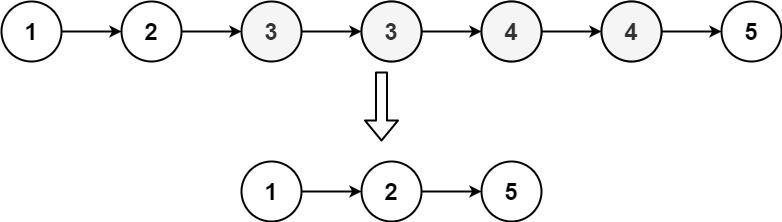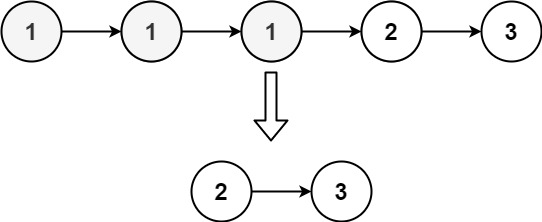## 82. Remove Duplicates from Sorted List II

Given the `head` of a sorted linked list, delete all nodes that have duplicate numbers, leaving only distinct numbers from the original list. Return the linked list sorted as well.

Example 1:```Input: head = [1,2,3,3,4,4,5]
Output: [1,2,5]
```

Example 2:```Input: head = [1,1,1,2,3]
Output: [2,3]
```

Constraints:

• The number of nodes in the list is in the range `[0, 300]`.
• `-100 <= Node.val <= 100`
• The list is guaranteed to be sorted in ascending order.

## Rust Solution

``````struct Solution;
use rustgym_util::*;

impl Solution {
let mut res: ListLink = None;
let mut stack: Vec<(i32, usize)> = vec![];
while let Some(node) = head {
let val = node.val;
match stack.last() {
Some(&(pval, count)) if pval == val => {
stack.pop();
stack.push((val, count + 1));
}
_ => {
stack.push((val, 1));
}
}
}
while let Some((val, count)) = stack.pop() {
if count == 1 {
}
}
res
}
}

#[test]
fn test() {
let head = list!(1, 2, 3, 3, 4, 4, 5);
let res = list!(1, 2, 5);